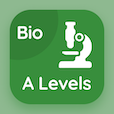Engineering Online Courses

Electronic Devices MCQs

Electronic Devices MCQ PDF - Topics

# JFET Biasing MCQ Quiz Online

Practice JFET Biasing Multiple Choice Questions (MCQ), JFET Biasing quiz answers PDF to learn electronic devices online course for electronic devices classes. Field Effect Transistors Multiple Choice Questions and Answers (MCQs), JFET Biasing quiz questions for online engineering associate's degree. "JFET Biasing MCQ" PDF Book: jfet biasing, metal oxide semiconductor field effect transistor, mosfet biasing test prep for online associate degree in engineering.

"If n-channel JFET with voltage Divider bias has a gate voltage of 3 V and a source voltage of 5 V then gate to source voltage will be" MCQ PDF: jfet biasing with choices 15 v, −2 v, 2 v, and −1.5 v for online engineering associate's degree. Learn jfet biasing quiz questions for merit scholarship test and certificate programs for job placement test.

## MCQs on JFET Biasing Quiz

MCQ: If n-channel JFET with voltage Divider bias has a gate voltage of 3 V and a source voltage of 5 V then gate to source voltage will be

15 V
−2 V
2 V
−1.5 V

MCQ: In a certain self-biased n-channel JFET circuit, if drain current is equals to 2mA and source resistance is equals to 1.0k Ω then gate to source voltage is equals to

2 V
−2 V
8 mV
−3 V

MCQ: If n-channel JFET with voltage divider bias has a gate voltage of 2 V and a source voltage of 10 V then gate to source voltage will be

8 V
−8 V
4 V
−4 V

MCQ: Most common type of JFET biasing is

voltage biasing
current biasing
self biasing
None of these

MCQ: A p-channel JFET requires a

positive gate to source voltage
negative gate to source voltage
positive drain to source voltage
negative drain to source voltage

### More Topics from Electronic Devices Course